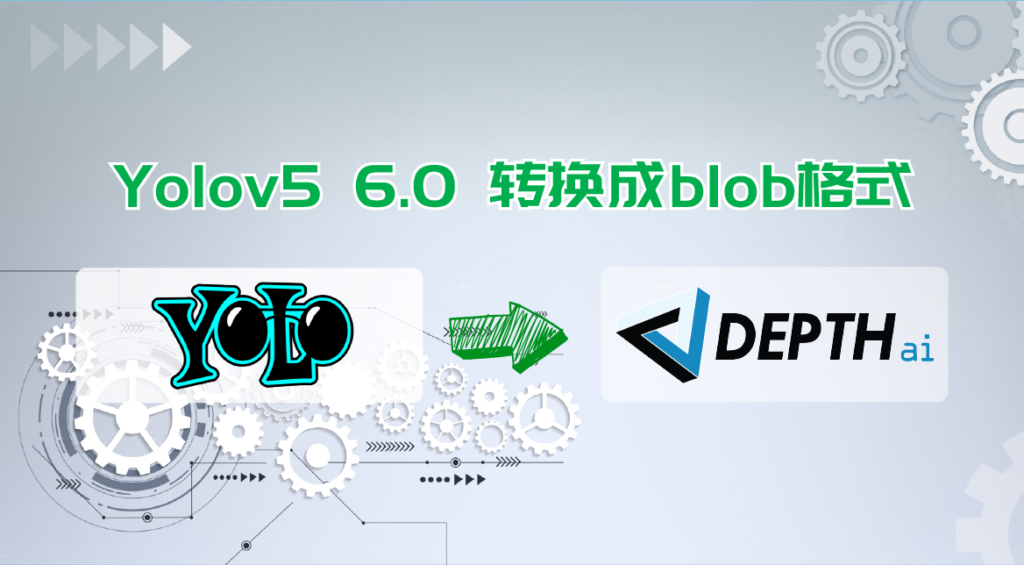+86-13951940532
contact@oakchina.cn

# Yolov5 6.0 转换成blob格式

## Yolov5 6.0 转换成blob格式depthai api 需要的 yolo 模型 输出格式为 B, N*(x, y, h, w, box_score, class_no_1, …,class_no_N), Cx, Cy,其中:

• B – 批量大小
• N – 检测框数量
• (x, y) – 检测框中心相对于单元格的坐标
• h, w – 框的原始高度和宽度，然后乘以相应的锚点得到绝对高度和宽度值
• box_score – 检测框置信度，在 [0,1] 范围内
• class_no_1, …, class_no_N – 概率分布，在 [0,1] 范围内，乘以置信度得到每个类的置信度
• Cx, Cy – 检测框索引

``````python export.py --simplify --opset 12 --include onnx --batch-size 1 --imgsz 640 --weights yolov5n.pt
``````

``````import onnx

conv_indices = []
for i, n in enumerate(onnx_model.graph.node):
if "Conv" in n.name:
conv_indices.append(i)

input1, input2, input3 = conv_indices[-3:]

sigmoid1 = onnx.helper.make_node(
'Sigmoid',
inputs=[onnx_model.graph.node[input1].output],
outputs=['output1_yolov5'],
)

sigmoid2 = onnx.helper.make_node(
'Sigmoid',
inputs=[onnx_model.graph.node[input2].output],
outputs=['output2_yolov5'],
)

sigmoid3 = onnx.helper.make_node(
'Sigmoid',
inputs=[onnx_model.graph.node[input3].output],
outputs=['output3_yolov5'],
)

onnx_model.graph.node.append(sigmoid1)
onnx_model.graph.node.append(sigmoid2)
onnx_model.graph.node.append(sigmoid3)

onnx.save(onnx_model, "yolov5n.onnx")

``````

## openvino 本地转换

#### onnx -> IR

``````mo.py --input_model yolov5n.onnx --scale 255 --reverse_input_channel --output "output1_yolov5,output2_yolov5,output3_yolov5"
``````

#### IR -> Blob

``````<path>/compile_tool -m yolov5n.xml \
-ip U8 -d MYRIAD \
-VPU_NUMBER_OF_SHAVES 6 \
-VPU_NUMBER_OF_CMX_SLICES 6
``````

#### blobconvert 代码 onnx -> Blob

``````blobconverter.from_onnx(
"yolov5n.onnx",
optimizer_params=[
"--scale=255",
"--reverse_input_channel",
"--output=output1_yolov5,output2_yolov5,output3_yolov5",
],
data_type="FP32",
shaves=6,
)
``````

#### blobconvert Cli

``````blobconverter --onnx yolov5n.onnx \
-dt FP32 -sh 6 -o . \
--optimizer-params "scale=255 --reverse_input_channel --output=output1_yolov5,output2_yolov5,output3_yolov5"
``````

## DepthAI 设置

``````import depthai as dai

pipeline = dai.Pipeline()
yolo = pipeline.create(dai.node.YoloDetectionNetwork)
yolo.setBlobPath("yolov5n.blob")
yolo.setConfidenceThreshold(0.5)
yolo.setNumClasses(80)
yolo.setCoordinateSize(4)
yolo.setAnchors([10,13, 16,30, 33,23, 30,61, 62,45, 59,119, 116,90, 156,198, 373,326])
"side80" : [0,1,2],
"side40" : [3,4,5],
"side20" : [6,7,8]
})
yolo.setIouThreshold(0.5)
``````
• input_size – 模型的输入形状,
• num_classes – Yolo 可以检测到的类数,
• coordinates – 坐标的个数（默认为 4）,
• anchors – yolo 锚点（位于 CFG 中的 [yolo] 层）,
• iou_threshold – iou 置信阈值,
• confidence_threshold – 检测到对象的置信阈值,
• anchor_masks – 锚掩码.

Tags: